A differential drive mobile robot has a movement based on two separately driven wheels placed on either side of the robot body. Thus, it is able to change its direction by varying the relative rate of rotation of its wheels. The mechanism is very simple since it does not require a steer. This makes this mobile robot very popular.

### 1. Dynamics

Assume that the robot is able to access and control the angular velocities of its wheels. Let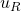and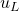denote the angular velocities of right and left wheels, respectively. Usage of stepper motors or closed-loop control of DC motors with optical encoders can achieve this. Thus, the inputs of the robots areand. We aim to represent the differential equation which characterizes the positions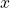and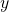of the center point and heading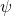of the body.

The robot has the knowledge of linear velocities of two points on its body owing to the ability to accessand. Figure 1 represents those velocities as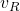and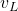. Those are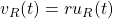and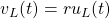(see: angular velocity). The angular velocity of the robot body is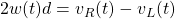(see: rigid body). Using the same identity, the linear velocity of the center point is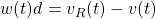. After algebraic manipulations, we derive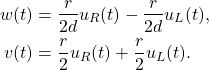Note that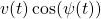andare the linear velocities of the center point in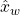and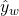directions, respectively. The differential drive mobile robot dynamics are given by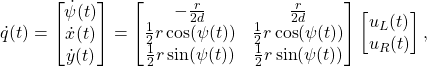where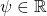is the heading direction,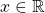is the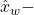position,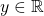is the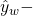position,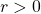is the radius of the wheels,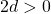is the distance between the wheels,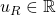is the angular velocity of the right wheel, and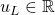is the angular velocity of the left wheel. The same dynamics can be represented in terms of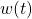and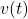as

(1)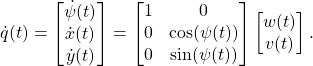### 2. Control

The system in (1) has a special structure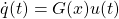where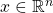,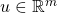, and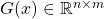. The systems of this form are \emph{nonholonomic control systems}. Although the system in (1) is controllable; yet, there exists no continuous feedback law that stabilizes the origin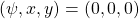. Please see the detailed discussion in Chapter 4.2 of .

Theorem (see Corollary 4.2 in ). The system in (1) cannot be asymptotically stabilized by a continuous feedback law.

Dr. Liberzon offers a switching control approach to stabilize the origin (or any set-point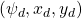) in .

### 4. References

 Liberzon, D. (2003). Switching in systems and control. Springer Science & Business Media.